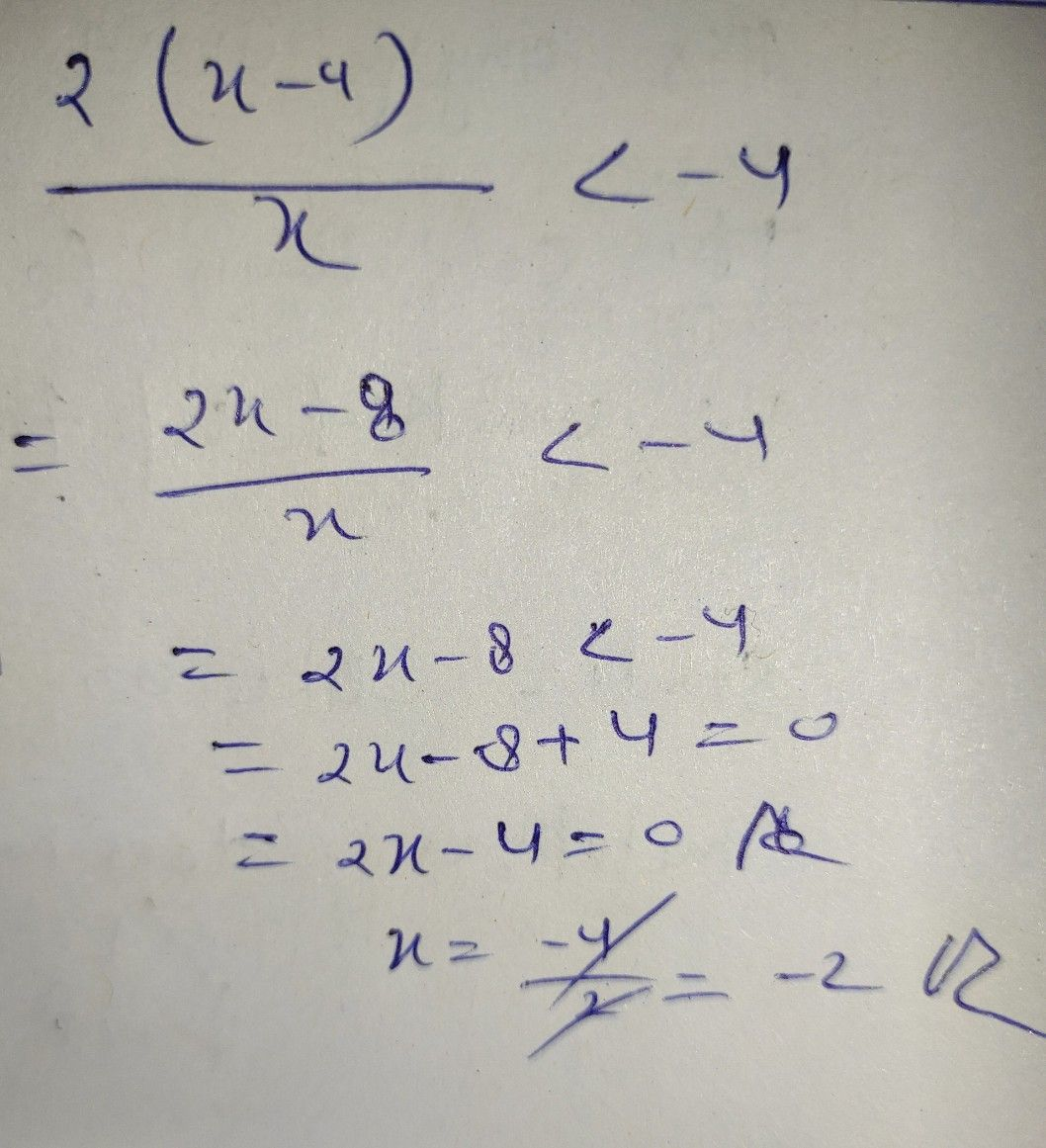Symbol
Problem5. Check each value $oy$ substituting into original equation and reject any extraneous $root/s$ $3$ Rational Inequality $\dfrac {2\left(x-4\right)} {x}<-4$ 1. Put the rational inequality in general form. $\dfrac {R\left(x\right)} {Q\left(x\right)}>0$ where $>$ can be replaced by $<,\leq$ $and$ $\geq$ $2$ $Vntθ$ the inequality into a single rational expression on the left side. $17$
1st-6th grade
Other
Search count: 112
SolutionQanda teacher - ASHU13696Student
i dont understand can you explain it to me sir?